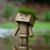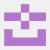## 一行简短的代码怎么也看不懂

JingleJin 发布于 2016/09/19 18:39

```li = [lambda:x for x in range(10)]
res = li()
print(res) #输出为9```

1```li = []
for x in range(10):
def _x():
return x
li.append(_x)

print(li())```

Python’s closures are late binding. This means that the values of variables used in closures are looked up at the time the inner function is called.

print一行才调用内部_x函数，而循环已结束，x = 9

00python里的lambda是捕获引用的，例如：

```>>> x = 234
>>> f = lambda:print(x)
>>> f()
234
>>> x = 456
>>> f()
456```

0
J

#### 引用来自“Kinegratii”的评论

```li = []
for x in range(10):
def _x():
return x
li.append(_x)

print(li())```

Python’s closures are late binding. This means that the values of variables used in closures are looked up at the time the inner function is called.

print一行才调用内部_x函数，而循环已结束，x = 9